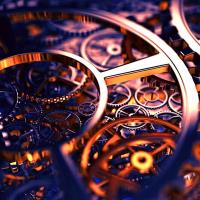Courses

# PPT: Finite Automata Notes | EduRev

## Computer Science Engineering (CSE): PPT: Finite Automata Notes | EduRev

The document PPT: Finite Automata Notes | EduRev is a part of the Computer Science Engineering (CSE) Course Theory of Computation.
All you need of Computer Science Engineering (CSE) at this link: Computer Science Engineering (CSE)
``` Page 1

1
Finite Automata
Page 2

1
Finite Automata
2
Finite Automaton (FA)
n Informally, a state diagram that comprehensively
captures all possible states and transitions that a
machine can take while responding to a stream or
sequence of input symbols
n Recognizer for “Regular Languages”
n Deterministic Finite Automata (DFA)
n The machine can exist in only one state at any given time
n Non-deterministic Finite Automata (NFA)
n The machine can exist in multiple states at the same time
Page 3

1
Finite Automata
2
Finite Automaton (FA)
n Informally, a state diagram that comprehensively
captures all possible states and transitions that a
machine can take while responding to a stream or
sequence of input symbols
n Recognizer for “Regular Languages”
n Deterministic Finite Automata (DFA)
n The machine can exist in only one state at any given time
n Non-deterministic Finite Automata (NFA)
n The machine can exist in multiple states at the same time
3
Deterministic Finite Automata
- Definition
n A Deterministic Finite Automaton (DFA)
consists of:
n Q ==> a finite set of states
n ? ==> a finite set of input symbols (alphabet)
n q
0
==> a start state
n F ==> set of accepting states
n d ==> a transition function, which is a mapping
between Q x ? ==> Q
n A DFA is defined by the 5-tuple:
n {Q, ? , q
0
,F, d }
Page 4

1
Finite Automata
2
Finite Automaton (FA)
n Informally, a state diagram that comprehensively
captures all possible states and transitions that a
machine can take while responding to a stream or
sequence of input symbols
n Recognizer for “Regular Languages”
n Deterministic Finite Automata (DFA)
n The machine can exist in only one state at any given time
n Non-deterministic Finite Automata (NFA)
n The machine can exist in multiple states at the same time
3
Deterministic Finite Automata
- Definition
n A Deterministic Finite Automaton (DFA)
consists of:
n Q ==> a finite set of states
n ? ==> a finite set of input symbols (alphabet)
n q
0
==> a start state
n F ==> set of accepting states
n d ==> a transition function, which is a mapping
between Q x ? ==> Q
n A DFA is defined by the 5-tuple:
n {Q, ? , q
0
,F, d }
4
What does a DFA do on
n Input: a word w in ?*
n Question: Is w acceptable by the DFA?
n Steps:
n Start at the “start state” q
0
n For every input symbol in the sequence w do
n Compute the next state from the current state, given the
current input symbol in w and the transition function
n If after all symbols in w are consumed, the current
state is one of the accepting states (F) then accept
w;
n Otherwise, reject w.
Page 5

1
Finite Automata
2
Finite Automaton (FA)
n Informally, a state diagram that comprehensively
captures all possible states and transitions that a
machine can take while responding to a stream or
sequence of input symbols
n Recognizer for “Regular Languages”
n Deterministic Finite Automata (DFA)
n The machine can exist in only one state at any given time
n Non-deterministic Finite Automata (NFA)
n The machine can exist in multiple states at the same time
3
Deterministic Finite Automata
- Definition
n A Deterministic Finite Automaton (DFA)
consists of:
n Q ==> a finite set of states
n ? ==> a finite set of input symbols (alphabet)
n q
0
==> a start state
n F ==> set of accepting states
n d ==> a transition function, which is a mapping
between Q x ? ==> Q
n A DFA is defined by the 5-tuple:
n {Q, ? , q
0
,F, d }
4
What does a DFA do on
n Input: a word w in ?*
n Question: Is w acceptable by the DFA?
n Steps:
n Start at the “start state” q
0
n For every input symbol in the sequence w do
n Compute the next state from the current state, given the
current input symbol in w and the transition function
n If after all symbols in w are consumed, the current
state is one of the accepting states (F) then accept
w;
n Otherwise, reject w.
5
Regular Languages
n Let L(A) be a language recognized by a
DFA A.
n Then L(A) is called a “Regular Language”.
n Locate regular languages in the
Chomsky Hierarchy
```Use Code STAYHOME200 and get INR 200 additional OFF Use Coupon Code
All Tests, Videos & Notes of Computer Science Engineering (CSE): Computer Science Engineering (CSE)

### Top Courses for Computer Science Engineering (CSE)## Theory of Computation

18 videos|44 docs|39 tests

### Top Courses for Computer Science Engineering (CSE)Track your progress, build streaks, highlight & save important lessons and more!

,

,

,

,

,

,

,

,

,

,

,

,

,

,

,

,

,

,

,

,

,

;